# Use of Simulation Models to Design, Optimization and Control of Wet Grinding Circuits

Mineral comminution is an important and expensive industrial process and modern comminution circuits are complex. There is considerable economic incentive to optimise circuit design and performance and to operate circuits at maximum efficiency, and these aims may be achieved by setting up mathematical models of the volts in a circuit on a digital computer and studying the behavior of simulated circuits.

## Mathematical Model of Comminution

For many years attempts were made to develop a mathematical description of the comminution process in terms of an energy-size reduction relationship. This was suitable for some purposes but was inadequate for simulation work in that the complete size distribution was not considered. In 1948 Epstein developed a statistical model of a breakage process which depended on two basic functions, Pn (y), the probability of breakage of a piece of size y in the nth step of a breakage process, and F(x,y), the distribution by weight of particles of size x less than or equal to y arising from breakage of-a unit mass of size y.

Broadbent and Calcott used the concepts of breakage, (B) , and selection, (S), functions in place of the distribution and probability functions of Epstein and wrote the equation

p = [B·S + I-S]v f

The value of a model depends upon the accuracy with which it may be used to describe a process and to predict the results of changes in that process. Seldom in dealing with real processes can models developed entirely from first principles be used for process analysis; assumptions are almost always necessary. Thus assumptions concerning the breakage function or the selection function do not detract from the value of the models provided that good agreement between predicted and observed mill behavior may be obtained over the operating range.

An anomaly exists in characterizing hydraulic classifications processes in that particles are separated in the classifiers on the basis of their hydraulic sizes, but the results of the process are normally assessed in terms of some measure of their physical size since for convenience screens are used.

An empirical model of a cyclone has been developed by Lynch and Rao which has been found suitable to describe the behavior of many plant cyclones. The basis of the model is as follows:

1. the coarse product is regarded as consisting of two components
a) particles which “short-circuit” to the product
b) particles which appear in the product due to classification
2. the “short-circuiting” particles are due to the water which appears in the underflow, and a corrected efficiency curve, shown as curve B in figure 1, describes the true classification process, and
3. the corrected efficiency curve may be.expressed as a dimensionless curve by equating the d50 (corrected) value to unity on the size axis. This concept is due to Yoshioka and Hotta.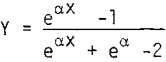## Circuit Optimization

The type of problems which occur in circuit optimization are:

1. for a given mill-cyclone system how many cyclones should be used, what should the vortex finder and spigot diameters be, and is there any advantage in using cyclones in series?
2. for a circuit containing more than one ball mill, how should the mills be arranged, what should be the classification conditions of the cyclones associated with each mill and if the mills are in series, what proportion of the total water should be added at each stage?

Step 1. Experimental data to be obtained from the circuit in its present form are as follows:

a) for known new ore and water additions, the size distributions and solids contents of all circuit streams and the mass flow rates of as many streams as possible, and
b) the cyclone dimensions, that is, vortex finder and spigot diameters, and the cyclone pressures relating to conditions in (a).

Step 2. Calculations to be made using these data are as follows:

a) mass balances at all points in the circuit
b) parameters of the rod and ball mills
c) parameters of the cyclones
Least squares and curve fitting techniques on a simulated circuit are normally necessary for these calculations.

Step 3. Operation of the ball mills in series involves putting all the fresh feed into the first ball mill and determining the equilibrium conditions of circuit operation for different water additions, number of cyclones and cyclone outlet dimensions using a computer program.

Step 4. When optimum conditions for primary circuit operation have been determined, the same procedure may be followed for secondary circuit operation using the calculated output from the primary circuit.

In circuit optimization the objective is definite and the problem is normally well defined in that tests may be carried out on the existing circuit and the parameters for use in the simulated circuit determined accurately. Design of a new circuit to augment or replace an existing circuit is essentially the same problem as circuit optimization in that the parameters of the existing circuit may be determined experimentally and the scale-up of breakage rates or their equivalent in the matrix model can be carried out on the basis of power consumption where it is desired to change the mill size.

If automatic control of a grinding circuit is to be possible, some means of detecting on-line the size of the particles in the circuit product is required. In developing this system, the view was taken that change in conditions in a grinding circuit results in changes in the circulating loads and the final product size so that if one cyclone stream is monitored and sufficient is known about the behavior of the cyclone, it should be possible to infer the final product size continuously by use of a cyclone as a sensor.

The use of the hydrocyclone as a size sensor was based on the following reasoning:

1. the calculated d50 (corr) of the cyclone operation is closely related to the minus 200 mesh in the cyclone overflow,
2. for a cyclone in an operating circuit, d50 (corr) may be calculated on-line if a mass flow metering system and a pressure sensor is installed on the cyclone feed line, and
3. the cyclone responds immediately to a change in feed conditions so it will detect a change in conditions immediately.

It was apparent that a corollary to the proposition that a cyclone is a suitable size sensor is that an inadequately controlled cyclone feed system will produce major fluctuations in the cyclone products. The advantage of the first control system was that these fluctuations had been markedly reduced. This improvement emphasized the importance of eliminating as far as possible variations in circuit performance due to incorrect mechanical conditions and this will normally eliminate the large, short term variations.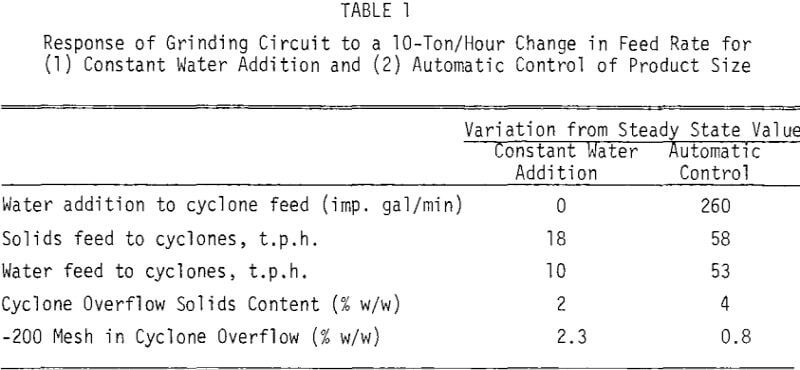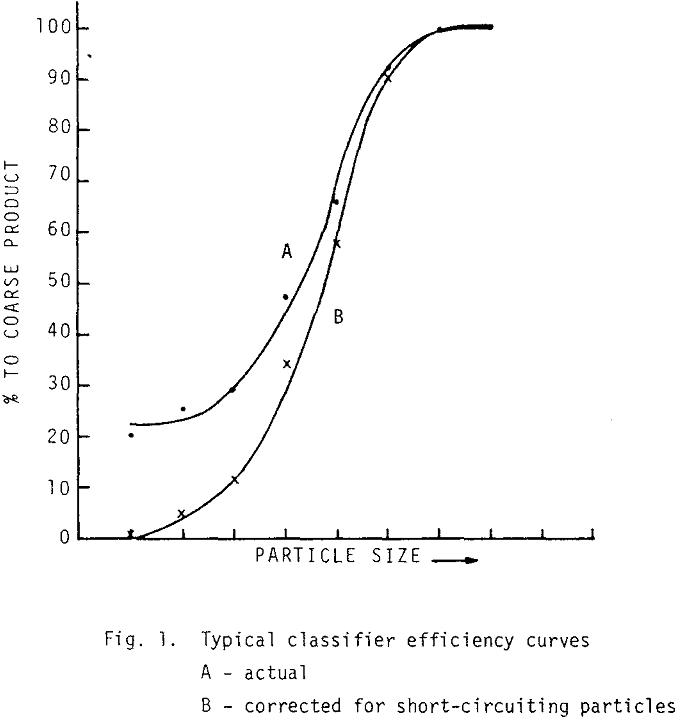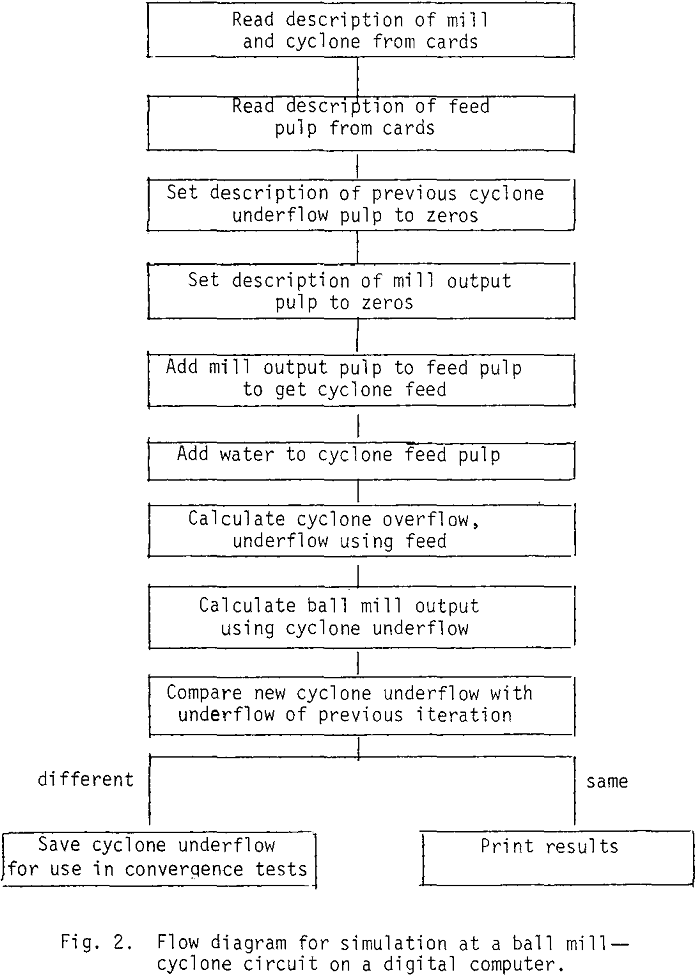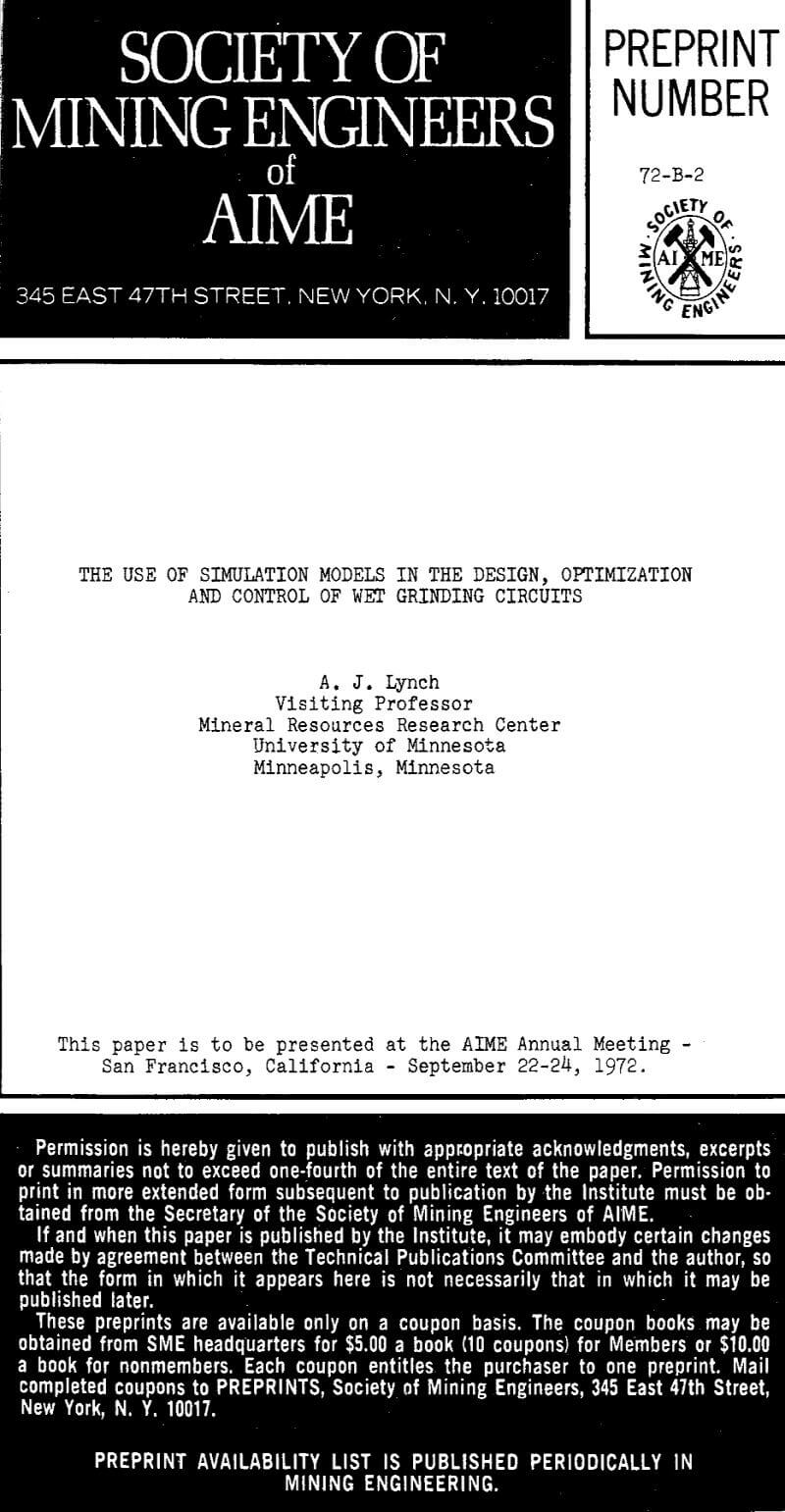By |2019-05-07T16:18:41-04:00May 2nd, 2019|Categories: Grinding|Tags: |Comments Off on Use of Simulation Models to Design, Optimization and Control of Wet Grinding Circuits

## BUY Laboratory & Small Plant Process Equipment

We have all the laboratory and plant equipment you need to test or build/operate your plant.

### ENTER our Mining Equipment' Store

We Sell EQUIPMENT for all types of Mineral Treatment PROCESSES and Laboratory Testing needs

## Do you need METALLURGICAL TESTING of your ORE?

View the Services we Provide

### 911Metallurgist Mineral Processing & Process Development Laboratory

We have a metallurgical test for every possible mineral type and treatment.

## Need ENGINEERING Services or Plant TROUBLESHOOTING?

We can IMPROVE ALL PLANTS / Mineral Processing Engineering & LABORATORY Ore Testing

### 911Metallurgy Engineering

Contact us for process engineering, metallurgical investigations, plant optimization, plant troubleshooting, needs. WE “FIX” METALLURGY.

I Need Ore Laboratory Testing